# Composite and Inverse Functions Worksheet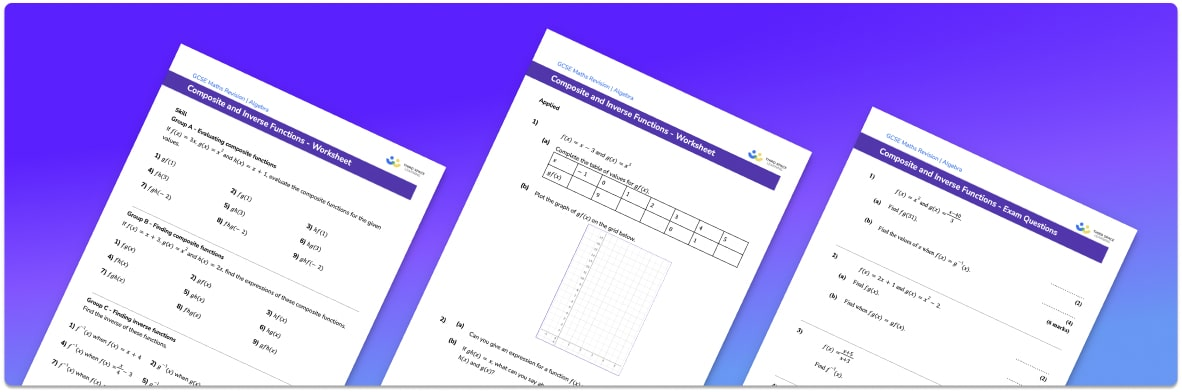• This field is for validation purposes and should be left unchanged.

You can unsubscribe at any time (each email we send will contain an easy way to unsubscribe). To find out more about how we use your data, see our privacy policy.

• Section 1 of the composite and inverse functions worksheet contains 27 skills-based questions, in 3 groups to support differentiation
• Section 2 contains 4 applied questions with a mix of worded problems and deeper problem solving questions
• Section 3 contains 4 foundation and higher level GCSE assessment questions in the form of exam questions
• Answers and a mark scheme for all questions are provided
• Follows variation theory with plenty of opportunities for students to work independently at their own level
• All questions created by fully qualified expert secondary maths teachers
• Suitable for GCSE maths revision for AQA, OCR and Edexcel exam boards

### Inverse and composite functions at a glance

Inverse functions are a way of reversing an original function.

The inverse of a function can be found for simple linear functions by using function machines. We can do this by finding the inverse operation for each stage in the function machine. It is important to have a strong understanding of inverse operations, for example the inverse of add 3 is subtract 3 and the inverse of square is square root. Care must be taken with the order of operations.

As functions become more complex, for example quadratic functions or when x is the denominator of an algebraic fraction, a more sophisticated method is required. This involves writing the original function as an equation in x and y, interchanging the variables and rearranging the equation to make y the subject.

This topic also covers composite functions. This is when two or more functions are applied in succession. Composite functions can be evaluated by substituting a value into the inner function, and then the answer is substituted into the outer function. Care must be taken to apply the functions in the correct order.

Inverse functions and composite functions can also be used to form and solve algebraic equations.

Looking forward, students can then progress to additional algebra worksheets, for example a factorising worksheet or a simultaneous equations worksheet.For more teaching and learning support on Algebra our GCSE maths lessons provide step by step support for all GCSE maths concepts.

## Related worksheets

Function Notation Worksheet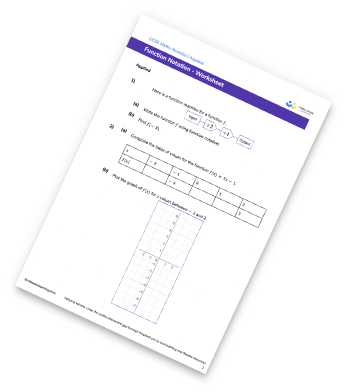Function Machines Worksheet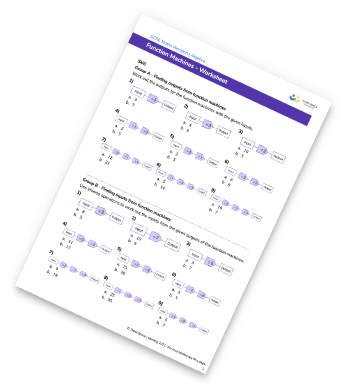Functions Worksheet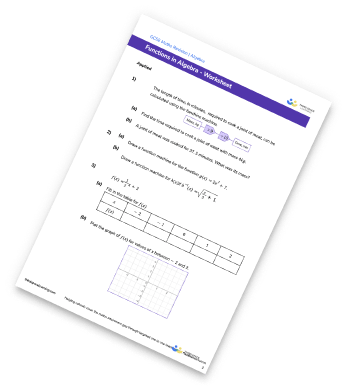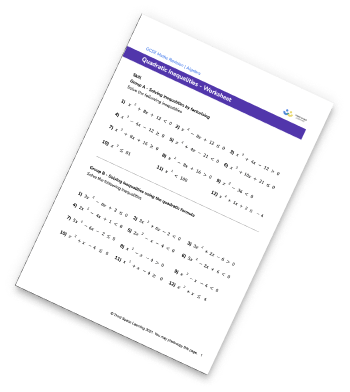## Do you have KS4 students who need more focused attention to succeed at GCSE?There will be students in your class who require individual attention to help them succeed in their maths GCSEs. In a class of 30, it’s not always easy to provide.

Help your students feel confident with exam-style questions and the strategies they’ll need to answer them correctly with our dedicated GCSE maths revision programme.

Lessons are selected to provide support where each student needs it most, and specially-trained GCSE maths tutors adapt the pitch and pace of each lesson. This ensures a personalised revision programme that raises grades and boosts confidence.

Find out more